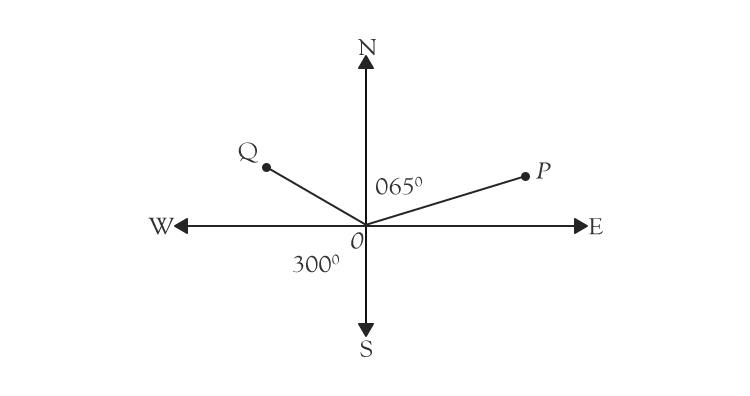## What is bearing angle and calculate between two Points

In geometry angles are measured regardless of direction or orientation as in trigonometry we measure angle by rotating anti clockwise. Here x-axis is (0) zero degree and y-axis is at 90 degree. So in this article we get familiar with What is bearing and calculate angle between two points. In GIS bearing angle is used for navigation or direction.

Bearing is a direction measured from north and it tracks angle in clockwise direction with north line which means north represents zero degree, east is 90 degrees, south is 180 degrees and west is 270 degrees.

Bearing angle at a point with respect to north at P if we measured clockwise then we see in gif is 065 degrees and at Q it is 300 degrees.A bearing is used to determine direction of one point relative to another point. You can see in  gif given below.β = atan2(X,Y),